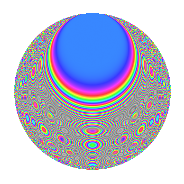# Properties

 Label 768.2.oLevel 768 Weight 2 Character orbit o Rep. character $$\chi_{768}(95,\cdot)$$ Character field $$\Q(\zeta_{8})$$ Dimension 112 Newform subspaces 2 Sturm bound 256 Trace bound 3

# Related objects

## Defining parameters

 Level: $$N$$ $$=$$ $$768 = 2^{8} \cdot 3$$ Weight: $$k$$ $$=$$ $$2$$ Character orbit: $$[\chi]$$ $$=$$ 768.o (of order $$8$$ and degree $$4$$) Character conductor: $$\operatorname{cond}(\chi)$$ $$=$$ $$96$$ Character field: $$\Q(\zeta_{8})$$ Newform subspaces: $$2$$ Sturm bound: $$256$$ Trace bound: $$3$$ Distinguishing $$T_p$$: $$7$$

## Dimensions

The following table gives the dimensions of various subspaces of $$M_{2}(768, [\chi])$$.

Total New Old
Modular forms 576 144 432
Cusp forms 448 112 336
Eisenstein series 128 32 96

## Trace form

 $$112q - 8q^{9} + O(q^{10})$$ $$112q - 8q^{9} + 16q^{13} + 8q^{21} - 16q^{25} - 16q^{33} + 16q^{37} + 8q^{45} - 8q^{57} + 80q^{61} + 8q^{69} - 16q^{73} + 96q^{85} - 16q^{93} - 32q^{97} + O(q^{100})$$

## Decomposition of $$S_{2}^{\mathrm{new}}(768, [\chi])$$ into newform subspaces

Label Dim. $$A$$ Field CM Traces $q$-expansion
$$a_2$$ $$a_3$$ $$a_5$$ $$a_7$$
768.2.o.a $$56$$ $$6.133$$ None $$0$$ $$-4$$ $$0$$ $$8$$
768.2.o.b $$56$$ $$6.133$$ None $$0$$ $$4$$ $$0$$ $$-8$$

## Decomposition of $$S_{2}^{\mathrm{old}}(768, [\chi])$$ into lower level spaces

$$S_{2}^{\mathrm{old}}(768, [\chi]) \cong$$ $$S_{2}^{\mathrm{new}}(96, [\chi])$$$$^{\oplus 4}$$$$\oplus$$$$S_{2}^{\mathrm{new}}(384, [\chi])$$$$^{\oplus 2}$$

## Hecke characteristic polynomials

There are no characteristic polynomials of Hecke operators in the database